# Monthly Archives

5 Articles

## Power BI Pro Tip: Pareto analysis with DAX / Power Pivot

Today’s post is a guest post by Michiel Rozema (https://www.linkedin.com/in/michielrozema). Thanks Michiel!

Dutch Data Dude Jeroen approached me with the question whether it would be possible to create a Pareto chart from a Power Pivot model, using DAX. Doing a Pareto analysis using Excel is easy and numerous ways of doing it can be found online, but Jeroen wanted to use DAX formulas and could not find the solution online. I’m always in for a challenge, so here we go…

A Pareto chart (https://en.wikipedia.org/wiki/Pareto_chart) is a combo chart containing a column chart for a certain value, sorted in descending order, and a line chart with the cumulative column values, expressed as a percentage. Like this: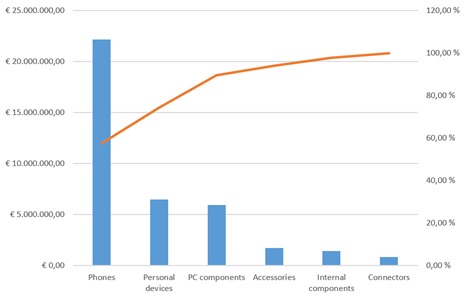The issue here is, of course, the cumulative percentage. It resembles a year-to-date total where we have months on the X-axis: for e.g. the month of May, the year-to-date total is the total for all months up to and including May. In the Pareto chart above, the percentage value for Accessories is the total of all product categories up to and including the Accessories category itself. There is no built-in DAX function for this, but as it turns out, a simple combination of a few DAX table functions does the trick; including a use of TOPN that I had not thought of before.

Let’s start with the data model. I have created a simple model with two tables, one for sales numbers and one for products: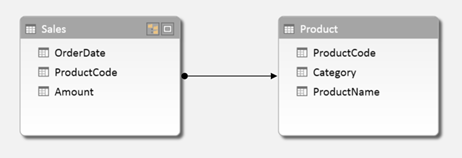We want to create a Pareto chart based on product categories, which is actually the chart shown above. For the column values in the chart, I create a basic calculated field:

For the cumulative percentage field, we need to calculate the cumulative total and divide that by the total amount for all categories. So let’s first create a calculated field for the latter one:

In this formula, ALL(Product[Category]) removes an existing filter from the Category column, therefore returning the result [TotalAmount] for all categories instead of only one.

Now it’s time to calculate the cumulative total. Let’s take the Accessories category as an example. To calculate the cumulative total for Accessories, we need to somehow determine that there are three categories placed to the left of Accessories, calculate their values, and add up the whole thing.

Remember that in the chart, the results for [TotalAmount] are shown in descending order. So we can say that for Accessories, we need to sum all categories for which [TotalAmount] is larger than the result for Accessories. If we had a Category table in our model with [TotalAmount] as a column, we could have made this calculation in a calculated column with a formula like the following:

However, we don’t have this column, [TotalAmount] cannot be a column either (we may want to add other tables to the model later on and to be able to filter the chart on customer segment, or year) and using calculated columns is not a good idea in general. So we need to take a different approach using calculated fields, and we cannot use EARLIER because we will not have a row context EARLIER can refer to.

To rephrase the cumulative total problem, we need to be able to pick some categories out of the whole list of categories based on the results of [TotalAmount]. There is a DAX function that can do this: TOPN. The obvious use of TOPN is to do calculation on for instance the top 10 customers, but in this case we will use a variable value of N in TOPN. Taking Accessories as an example again, we need to calculate the total amount for the top 4 categories. But to do that, we need to determine that Accessories is the number 4 category when it comes to [TotalAmount]. For this, we use another table function, RANKX. So we first create the calculated field below:

What does RANKX do? To quote the Power Pivot tool tip, it ‘Returns the rank of an expression in the current context in the list of values for the expression evaluated for each row in the specified table’. So, our calculation evaluates [TotalAmount] in the current context (in our example, the Accessories category), then loops through the rows of ALL(Product[Category]), which is a list of all categories (remember that ALL is a table function, and we need to use ALL because of the current context), and evaluates [TotalAmount] for each category. It then returns the rank of the result for Accessories in the list for all categories. Below is the list of results of [TotalAmount] for all categories: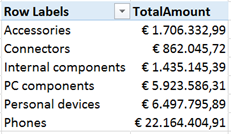When we sort the list in descending order, we can see that indeed, Accessories is the 4th category: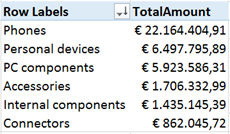With the rank, we can now calculate the cumulative total using the TOPN function:

The calculated table we use in this SUMX statement:

returns, in our example Accessories category, the four categories with the largest value of [TotalAmount]. The SUMX itself sums the [TotalAmount] values of these four categories.

Now, the only thing left to do is to calculate the Pareto percentage:

In the chart, we sort on the [TotalAmount] field used for the columns, and put [Pareto%Category] as a line chart on the secondary axis.

Creating a Pareto analysis on the Product level works exactly the same, obviously, the only difference is that we have to take care of two columns that can filter the products, [ProductCode] and [ProductName]. The calculated fields are below:

Here’s the Pareto chart with the large number of products:Just for fun, we can add categories to the X-axis and have many Pareto charts in one. I don’t really think this makes sense, but it’s nice that it works and returns the right percentages in each category. It works this way because we used the right ALL statement in our calculations.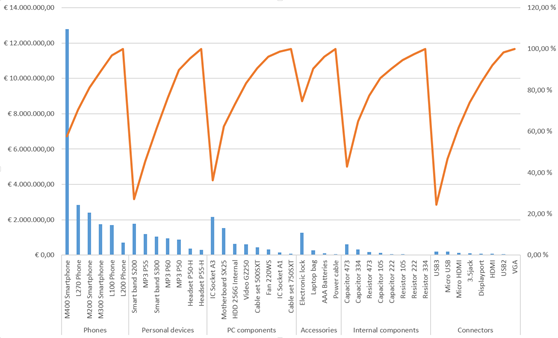So, creating a Pareto chart with mostly DAX can be done. And the combination of RANKX and TOPN turns out to be a very powerful one, which will certainly prove useful in other situations.

## Webinar on Azure Machine Learning

Last week I did a live webinar on Azure Machine Learning. The webinar can serve as a introduction into the subject of machine learning, data mining, predictive analytics as well as Microsoft’s solution for it: Azure Machine Learning.

Watch the recording here (in Dutch, sorry).

## Power BI Pro Tip: making date / time calculations work (Time Intelligence)

Ever so often I get asked how to do a year-over-year, quarter-over-quarter, month-over-month or year-vs-year calculation in Power BI. In most cases people would like to create a KPI to measure a certain periods performance compared to another. Power BI (specifically DAX) provides great functions for this; the Time Intelligence functions. In this scenarios PREVIOUSMONTH, PREVIOUSYEAR, SAMEPERIODLASTYEAR are used most. However, there are some frequent mistakes that result in errors when using these functions:

1) You will need to have a Date table in your model. Technically you do not need one, but you need to make sure the column you use for the time based calculations contains only unique values/dates. This is often not the case with sales happening more than once a day! Once you have the date table in the model, make sure to create a relationship between your facts date (for example sales date) and the date table.

2) The time intelligence functions should really be used as measures; not as calculated columns. This means their position in the Excel PowerPivot 2013 screen is under the horizontal line, not in the columns above.

3) Time intelligence functions work best when using totals, averages or other aggregated info.

Here are some examples. I will use ‘Date'[Date] as the reference to my date column in my date table. Also, for a best practice I split the calculation in two parts: the first part just calculates the total sales, while the other calculations refer to that base calculation.

Note that the last one uses SAMEPERIODLASTYEAR which is more flexible as it will select the same day in the previous year or the same month in the previous year depending on the selection the user makes in the tables/graphs. This is however not always what you want; so you can make it more specific by using PREVIOUSYEAR / PREVIOUSMONTH etc.

You could also use DATEADD to be even more flexible:

Notice by the way that I tend to use the short-hand notation to prevent me from having to type CALCULATE all the time (yes I am lazy :)). Here is an example of the two ways to get the sales for the previous year, the first line is the short-hand, the second is the more elaborate but not less correct option:

Hope this helps!

I had to figure out recently how to load multiple JSON files using Power Query. It turned out to be less easy than expected, so I figured it is worth blogging about…

The scenario: I have multiple JSON files sitting in a container in Azure Blob Storage; I would like to load them all into a data model for use in Power BI. I am assuming all the files you want to load are in one container. My solution will not work for multiple containers.

I will be using Power Query for this, from the Power BI Designer. You could do the same using Power Query in Excel.

First, let’s connect to the blob storage. This part is easy. Just click Get Data à More in the Power BI Designer and then select ‘Azure’ and then choose Microsoft Azure Blob Storage and click Connect: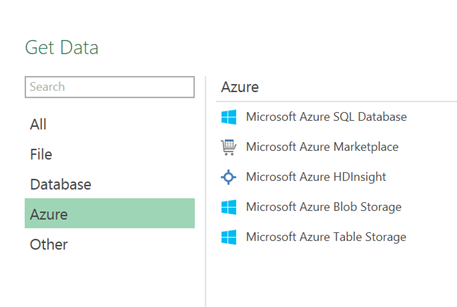In Excel, navigate to the Power Query tab, select From Azure à From Microsoft Azure Blob Storage: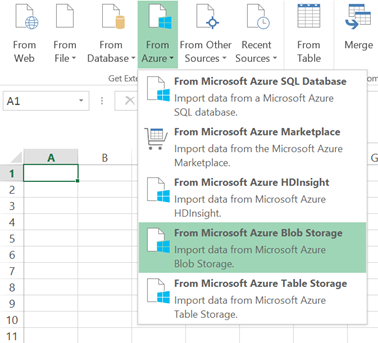You will need to enter your Azure Storage account name and key. Next, you will see a list of containers in the blob storage. Select the container the data is in and choose Edit: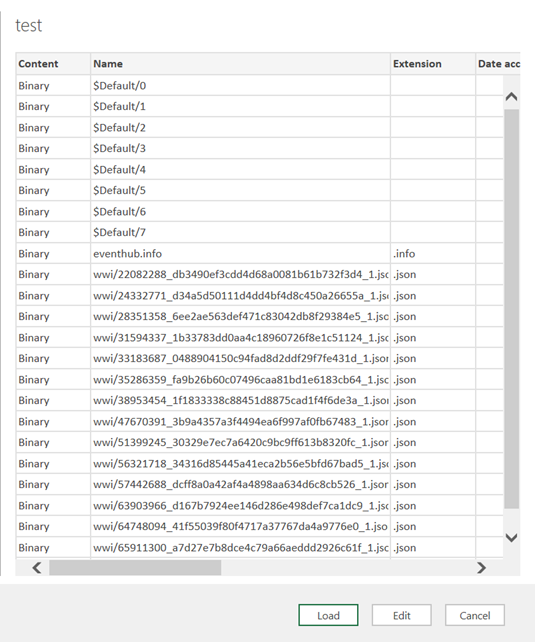What we will need to do is create a function that loads the JSON files. To do this we use an approach similar to loading multiple Excel or CSV files (see here and here respectively): first we just load one file and then we convert it into a function which we will call for all files we want to load.

So first, click on ‘Binary’ in the first column for one of the rows representing a JSON file. You will a one column table listing all records in the JSON file (the exact number of rows changes with the length of the JSON file):What you want to do is convert the records into a table by clicking on the button: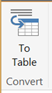You will probably see a ‘to Table’ dialogue, allowing customization of the conversion; for JSON you normally should not have to change the defaults, so click OK.

Next step is to expand the resulting Column1 to see some actual data. To do this click the expand button to the right of the column header and click OK (I deselected the ‘use original column name as prefix’ option):And voila: a nice looking table of the records in this JSON file: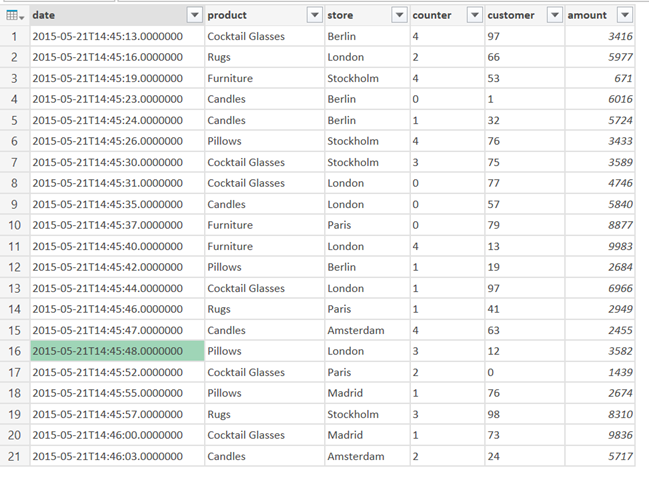We are not done however; this was the easy part. Remember we need to create a function that will enable us to iterate over multiple files.

To start editing the code hop over to the Advanced Editor (ViewàAdvanced Editor). Your code should look something like this: (Your last line will be different from mine since it is dependent on the contents of the JSON)

First, we will need to wrap this in a function, so add this line at the top:

Then, add this at the bottom:

We need to edit the line that defines ‘contents’ to look like this:

Your code should look like this:

Click ‘Done’ and give the query a descriptive name (I suggest naming it the same as the function: LoadJSON)… pfew, that was not too bad right? So now, let’s use this function on all our JSON files. Let’s do the same as we did at the start; connect to the blob and stop at the screen where you have a list of files in the container: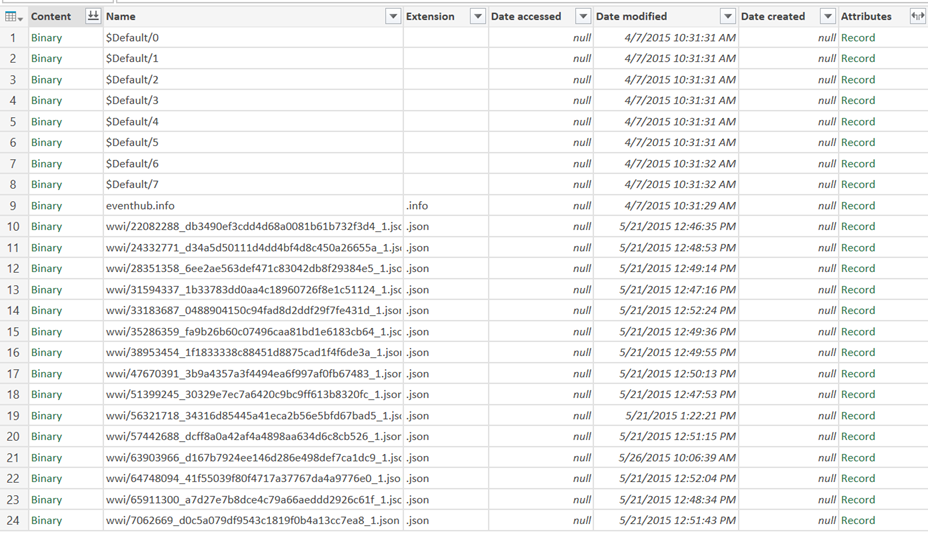Since we only can apply the function to JSON files, my first step is to filter on the Extension being just ‘.json’: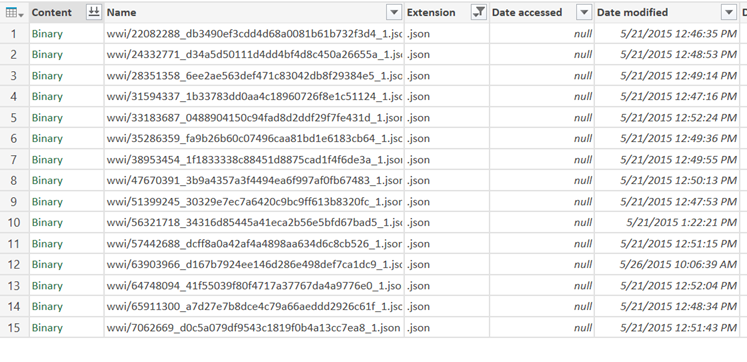Then, we need to get rid of all the columns except Name and Folder Path. To do this, select the columns to keep and choose Remove Other Columns.

Now, let’s call the function and pass in the path and name parameters. Insert a custom column (Add Column à Add Custom Column) with the following setting:

Then, we need to expand the resulting custom column by clicking on the little expand button again: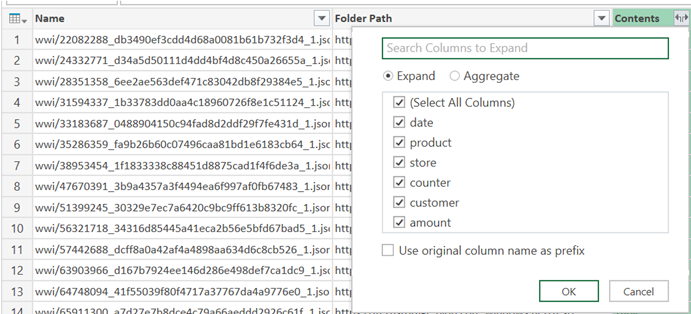Click ‘OK’. Now you have all the contents visible. To clean up lets delete the Name and Folder Path column since we do not need them anymore. Since this is JSON you will probably want to fix data types before reporting on this.

And…. You’re done, how cool is this?